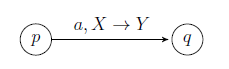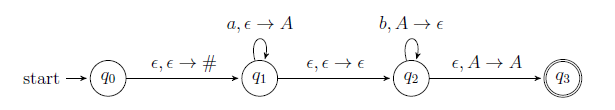# GATE | GATE CS 2021 | Set 1 | Question 61

In a pushdown automaton P=(Q,Σ,Γ,δ,q0,F), a transition of the form,where p,q∈Q, a∈σ∪{ϵ}, X,Y,∈Γ∪{ϵ}, represents

`(q,Y) ∈ δ(p,a,X) `

Consider the following pushdown automaton over the input alphabet Σ={a,b} and stack alphabet Γ={#,A}.The number of strings of length 100 accepted by the above pushdown automaton is ___________ .
(A) 50
(B) 100
(C) 55
(D) 45

```L = {anbm| n>m & n+m=100}